• 对二叉排序树进行中序遍历
2021-02-26 22:19:11
#include <iostream>
#include <cstdio>
#include <queue>
using namespace std;
struct TreeNode{
int data;
TreeNode *leftchild;
TreeNode *rightchild;
};

TreeNode* Insert(TreeNode* root, int x){//二叉排序树建树
if(root == NULL){
root = new TreeNode();
root->data = x;
root->leftchild = NULL;
root->rightchild = NULL;
}else if(x < root->data){
root->leftchild = Insert(root->leftchild,x);
}else{
root->rightchild = Insert(root->rightchild,x);
}
return root;
}
void InOrder(TreeNode* root){//二叉排序树中序输出升序所以叫排序树
if(root != NULL){
InOrder(root->leftchild);
printf("%d\n",root->data);
InOrder(root->rightchild);
}
return;
}
int main(){
int n;
while(scanf("%d",&n) != EOF){
TreeNode* root = NULL;
while(n--){
int x;
scanf("%d",&x);
root = Insert(root,x);
}
InOrder(root);
}
return 0;
}


更多相关内容
• 建立一位数组，然后以一位数组为例进行二叉排序树中序遍历
• 二叉搜索树（Binary Search Tree），（又：二叉搜索树，二叉排序树）它或者是一棵空树，或者是具有下列性质的二叉树： 若它的左子树不空，则左子树上所有结点的值均小于它的根节点的值； 若它的右子树不空，则右子树...

# 二叉搜索树的概念

二叉搜索树（Binary Search Tree），（又：二叉搜索树，二叉排序树）它或者是一棵空树，或者是具有下列性质的二叉树： 若它的左子树不空，则左子树上所有结点的值均小于它的根节点的值； 若它的右子树不空，则右子树上所有结点的值均大于它的根结点的值； 它的左、右子树也分别为二叉排序树。二叉搜索树作为一种经典的数据结构，它既有链表的快速插入与删除操作的特点，又有数组快速查找的优势；所以应用十分广泛，例如在文件系统和数据库系统一般会采用这种数据结构进行高效率的排序与检索操作。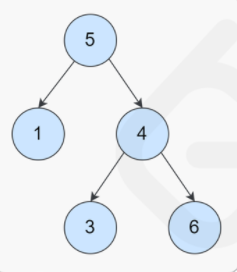图1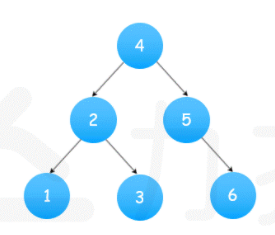图2

类似图1，此处3比5小，不是二叉搜索树，中序遍历得出的序列为[1, 5, 3, 4, 6]可以看出，并不是逐个递增的。图2是二叉搜索树，中序遍历得出的序列[1, 2, 3, 4, 5, 6]

# 二叉搜索树的性质

二叉树由于左子树的结点的值均小于根节点的值，右子树的结点的值均大于根节点的值。所以二叉搜索树可以根据中序遍历，转换成值递增的一维数组。 因此大部分的二叉搜索树的题目都可以先直接使用中序遍历，然后进行相应的操作。

中序遍历的方式可以递归，也可以迭代，迭代写法方便对序列进行操作，所以尽量使用迭代中序遍历。写法可以参考往期博客。

https://blog.csdn.net/weixin_42835209/article/details/115321081?spm=1001.2014.3001.5501

# 二叉搜索树相关leetcode题解

## leetcode-98. 验证二叉搜索树

思路：根据中序遍历把二叉树转换成List，逐个比较是否依次递增。利用了二叉搜索树的特性，方法较为暴力。

import java.util.ArrayList;
import java.util.List;

import 树.TreeNode;

public class IsValidBST {

public static void main(String[] args) {
TreeNode root1 = new TreeNode(1);
TreeNode root2 = new TreeNode(1);
//		TreeNode root3 = new TreeNode(6);
//		TreeNode root4 = new TreeNode(3);
//		TreeNode root5 = new TreeNode(7);

root1.left = root2;
//		root1.right = root3;
//		root3.left = root4;
//		root3.right = root5;
IsValidBST ivbst = new IsValidBST();

System.out.println(ivbst.isValidBST(root1));
}
List<Integer> list = new ArrayList<Integer>();
public List<Integer> midOrderTraversal(TreeNode root){
if(root == null) {
return list;
}
midOrderTraversal(root.left);
midOrderTraversal(root.right);
return list;
}

public boolean isValidBST(TreeNode root) {
if(root == null) {
return true;
}
List<Integer> arr = midOrderTraversal(root);
for(int i=1;i<arr.size();i++) {
if(arr.get(i) <= arr.get(i-1)) {
return false;
}
}
return true;
}
}

## leetcode-99. 恢复二叉搜索树

思路：1. 根据中序遍历，把树转换成List。2. 根据List找到对应的x和y，x为第一个比后面的数大的数字，y为最后一个比x小的数。交换树中对应x结点和y结点的值。


import java.util.ArrayList;
import java.util.List;
import java.util.Stack;

import 树.TreeNode;

public class RecoverTree {

public static void main(String[] args) {
TreeNode root1 = new TreeNode(1);
TreeNode root2 = new TreeNode(3);
TreeNode root3 = new TreeNode(2);

root1.left = root2;
root2.right = root3;
RecoverTree rt = new RecoverTree();
rt.recoverTree(root1);
}

public void recoverTree(TreeNode root) {
List<Integer> nums = new ArrayList<Integer>();
inorder(root, nums);
int [] swapped = findTwoSwapped(nums);
recover(root, 2, swapped, swapped);

}

public void inorder(TreeNode root, List<Integer> nums) {
if(root == null) {
return;
}
inorder(root.left, nums);
inorder(root.right, nums);
}

public int[] findTwoSwapped(List<Integer> nums) {
int n = nums.size();
int x = -1, y = -1;
for(int i=1;i<n;i++) {
if(nums.get(i) < nums.get(i-1)) {
y = nums.get(i);
if(x == -1) { // 只记录第一次
x = nums.get(i-1);
}else {
break;
}
}
}
return new int[] {x, y};
}

public void recover(TreeNode root, int count, int x, int y) {
if(root != null) {
if(root.val == x || root.val == y) {
root.val = root.val == x?y:x;
if(--count == 0) { // 找到再减count
return;
}
}
recover(root.right, count, x, y);
recover(root.left, count, x, y);
}
}
}

## leetcode-230. 二叉搜索树中第K小的元素

思路：把二叉树转换成List，按索引取数字，第k小对应的索引为k-1。

import java.util.ArrayList;
import java.util.List;

import 树.TreeNode;

public class KthSmallest {

public static void main(String[] args) {

}

public int kthSmallest(TreeNode root, int k) {
List<Integer> nums = new ArrayList<>();

inorder(root, nums);
return nums.get(k-1);
}

public void inorder(TreeNode root, List<Integer> nums) {
if(root == null) {
return;
}
inorder(root.left, nums);
inorder(root.right, nums);
}
}

## leetcode-二叉搜索树中的中序后继

思路：用迭代写法的中序遍历，定义一个flag初始化为false，代表是否找到p。如果找到p，则置flag为true，则下一次迭代时得到的node则为p的后继。由于中序遍历取数都是在栈中弹栈才取的，所以需要在弹栈的时候进行flag的判断和修改。

import java.util.ArrayList;
import java.util.List;
import java.util.Stack;

import 树.TreeNode;

public class InorderSuccessor {
public TreeNode inorderSuccessor(TreeNode root, TreeNode p) {
if(root == null) {
return null;
}

// 迭代中序遍历
Stack<TreeNode> stack = new Stack<>();
boolean flag = false;
while(root!=null || !stack.empty()) {
while(root!=null) {
stack.push(root);
root = root.left;
}
TreeNode node = stack.pop();
if(flag == true) {
return node;
}
// 判断p的值是否相等，如果相等，则设置为true,获得下一次遍历的值
if(node.val == p.val) {
flag = true;
}
if(node.right != null) {
root = node.right;
}
}
return null;
}

}


## leetcode-最接近的二叉搜索树值II

思路：先实现中序遍历，用一个map记录<差值，原值>，在java中转换成TreeMap进行升序排序。得到差值小的排在前面，则根据k得到前k个差值最小的数字，即最接近的数字进行返回。

import java.util.ArrayList;
import java.util.Collection;
import java.util.Collections;
import java.util.Comparator;
import java.util.HashMap;
import java.util.List;
import java.util.Map;
import java.util.PriorityQueue;
import java.util.Queue;
import java.util.Set;
import java.util.Stack;
import java.util.TreeMap;

import 树.TreeNode;

public class ClosestKValues {

List<Integer> closestKValues(TreeNode root, double target, int k){
List<Integer> res = new ArrayList<Integer>();
if(root == null) {
return res;
}
Stack<TreeNode> stack = new Stack<>();
Map<Double, Integer> map = new HashMap<Double, Integer>();
while(!stack.empty() || root != null) {
while(root != null) {
stack.push(root);
root = root.left;
}
TreeNode node = stack.pop();
map.put(Math.abs(node.val-target), node.val);
if(node.right != null) {
root = node.right;
}
}

TreeMap<Double, Integer> sorted = new TreeMap<>(map);
System.out.println(sorted.toString());
List<Integer> arr = new ArrayList<Integer>(sorted.values());
for(int i=0;i<k;i++) {
}
Collections.sort(res);
return res;
}

}


# 总结

总而言之，只要是二叉搜索树的题目，都可以实现中序遍历先。中序遍历可以先额外写一个递归的中序遍历函数返回各种参数，也可以直接用迭代的中序遍历直接在迭代体中进行一系列操作。主要是要掌握各种数据结构容器的使用，并深刻理解树的遍历的写法。

展开全文数据结构 二叉树 leetcode
•二叉树
• 中序遍历二叉排序树 输入一整数序列，建立二叉排序树，然后中序遍历。 输入说明 输入第一行为整数的个数n，第二行是具体的n个整数。 输出说明 建立二叉排序树，然后输出中序遍历的结果。 输入样例 5 1 6 5 9 8 ...数据结构
• 给你一个二叉树的根节点 root ，判断其是否是一个有效的二叉搜索树。 有效 二叉搜索树定义如下： 节点的左子树只包含 小于 当前节点的数。...算法：树中序遍历的顺序为左根右，根据二叉树搜索树的性质，如果一棵树满

给你一个二叉树的根节点 root ，判断其是否是一个有效的二叉搜索树。

有效 二叉搜索树定义如下：

节点的左子树只包含 小于 当前节点的数。
节点的右子树只包含 大于 当前节点的数。
所有左子树和右子树自身必须也是二叉搜索树。

示例 1：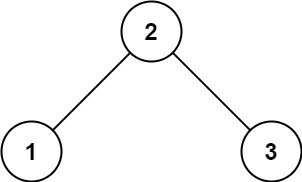输入：root = [2,1,3]
输出：true
示例 2：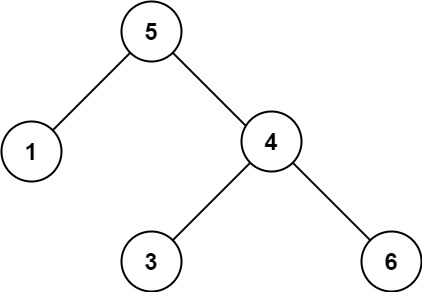输入：root = [5,1,4,null,null,3,6]
输出：false
解释：根节点的值是 5 ，但是右子节点的值是 4 。

算法：树中序遍历的顺序为左根右，根据二叉树搜索树的性质，如果一棵树满足二叉搜索树，它的中序遍历结果一定是升序的；
java栈实现

class Solution {
public boolean isValidBST(TreeNode root) {
double inorder = -Double.MAX_VALUE;

while (!stack.isEmpty() || root != null) {
while (root != null) {
stack.push(root);
root = root.left;
}
root = stack.pop();
// 如果中序遍历得到的节点的值小于等于前一个 inorder，说明不是二叉搜索树
if (root.val <= inorder) {
return false;
}
inorder = root.val;
root = root.right;
}
return true;
}
}

展开全文算法 数据结构
• 文章目录【1】代码：①Node节点②BST binarySortTree二叉排序树：③ 测试代码：【2】测试结果： 【1】代码： ①Node节点 package algorithm.tree.binarySortTree_BST; public class Node { int val; Node left; ...

# 【1】代码：

## ①Node节点

package algorithm.tree.binarySortTree_BST;

public class Node {
int val;
Node left;
Node right;

public Node(int val) {
this.val = val;
}

@Override
public String toString() {
return val+"";
}
if(node == null)
return;
if (node.val<this.val){
if (this.left == null){
this.left = node;
}else{
//向左递归添加
}
}else{
if (this.right == null){
this.right = node;
}else{
//右节点不为空就递归添加
}
}
}
}



## ②BST binarySortTree二叉排序树：

package algorithm.tree.binarySortTree_BST;

public class BTS {
//根节点
Node root;

//添加
if (root == null){
root = n;
}else{
}
}
//给外部中序遍历的接口
public void show(){
midOrder(root);
}
//中序遍历
private void midOrder(Node n){
//左边
if (n == null)
return;
if (n.left != null){
midOrder(n.left);
}
//打印
System.out.println(n);
//右边
if (n.right != null){
midOrder(n.right);
}
}

}



## ③ 测试代码：

package algorithm.tree.binarySortTree_BST;

public class Test {
public static void main(String[] args) {
int[] arr = {4, 6, 2, 12, 45, 5, 123, 6, 2};
BTS tree = new BTS();
for (int t:arr) {
}
tree.show();
}
}



# 【2】测试结果：

1. 待测试数据：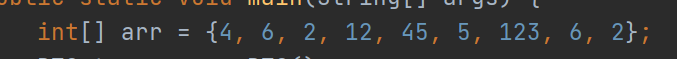2. 结果：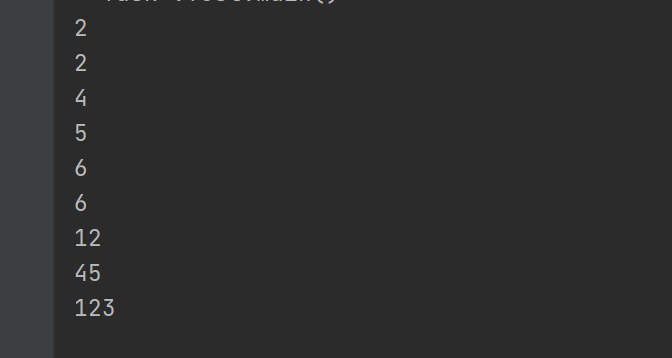展开全文算法
• 一、题目描述 给你一个二叉树的根节点 root ，判断其是否是一个有效的二叉搜索。 有效 二叉搜索定义如下： 节点的左子树只包含 小于 当前节点的数。 节点的右子只包含 大于 ...中序遍历 + 递归 当前节点是否大于leetcode 数据结构
• 版权声明：本文为博主原创文章，遵循 CC 4.0 BY-SA 版权协议，转载请附上原文出处链接和本声明。 本文链接：...（我们一般在书中试题中见到的是二叉树，但并不意味着所有的都是二...
• 对二叉排序树中序遍历(非递归不用栈队列） 找到这棵树的中序遍历的第一个节点 相当于找这课二叉树的最小值 BstNode* First(BstNode* ptr)//找到这棵树的中序遍历的第一个节点 { while (ptr != nullptr &&...二叉树 算法 链表 数据结构
• * 二叉排序树BST的插入和中序遍历 * 排序树的中序遍历输出序列是递增的 **/ #include<iostream> using namespace std; #define DataType int #define test_length 12 DataType test_data[test_length]={5,...c++ 数据结构 二叉树
• 实现要求： ...该二叉树类提供方法进行节点的追加(追加节点时，构建二叉排序树)，并提 供中序遍历二叉树节点的方法。 BinaryTreeNode类 public class BinaryTreeNode { private int data; priv...数据结构 java 二叉树
• } //查看树中的值 System.out.println("二叉排序树中序遍历："); bst.midShow(); System.out.println(); System.out.println("============="); Node node = bst.search(10); System.out.println(node.value); Node...
• !... 我看书上说“二叉排序树中序遍历结果是递增序列”，然后我随便写了几个数，生成二叉排序树进行中序遍历，结果如上图，它不是递增序列啊？？？是我知识上有什么漏洞吗？求教啊~~
• 概述： ...点，这个时候我们就引入了二叉排序树。 树结构查找是将查找表按照某种规律建成树结构。因为建构的树结构是按某种规律建立的，因此查找 过程也遵循这种规律，可以获得较高的查找效率。 1、...
• 二叉排序树创建、中序遍历（由小到大）、交换左右子树输出（由大到小），完整C++实现代码，在Clion中编译通过。 #include "stdio.h" #include "stdlib.h" #include "malloc.h" //...C++ 交换左右子树
• （2） 建立一棵二叉排序树，然后进行中序遍历，并实现动态查找。(输入零为空） 头文件：1.h #include &lt;iostream&gt; #include &lt;malloc.h&gt; #include &lt;string.h&gt; #define TRUE...
• 根据输入数据建造二叉排序树，并中序遍历，从而输出结果
• 恢复二叉搜索 二叉搜索中的两个节点被错误地交换。 请在不改变其结构的情况下，恢复这棵。 示例1: 输入: [1,3,null,null,2] 1 / 3 \ 2 输出: [3,1,null,null,2] 3 / ...LeetCode
• 二叉排序树的定义 二叉排序树，也称二叉查找树。二叉排序树或者是一棵空树，或者是一颗具有以下特性的非空二叉树： ...右子树结点值，二叉树中序遍历可得到一个递增的有序序列。 二叉排序树的创建...数据结构
• 给定一个二叉树，判断它是否是合法的二叉查找(BST) 一棵BST定义为： 节点的左子树中的值要严格小于该节点的值。节点的右子中的值要严格大于该节点的值。左右子树也必须是二叉查找。一个节点的也是二叉查找...算法
• 【算法步骤】 扫描字符序列，读入字符ch. ...创建二叉链表如图所示： 代码如下： #include <iostream> using namespace std; typedef struct BiNode { char date; //二叉链表的定义 struct BiNode *lchi链表 二叉树 算法 数据结构 c++
• 针对这一数据结构的遍历问题主要有四种，前序遍历、中序遍历、后序遍历、层序遍历，我们主要说明一下二叉树前序、中序、后序的递归方式代码模板。 基本思想 前序遍历：根结点 —> 左子树 —> 右子 中序...java 算法 数据结构
• 将有序数组转换为二叉搜索中序遍历，递归） https://leetcode-cn.com/problems/convert-sorted-array-to-binary-search-tree/ 将一个按照升序排列的有序数组，转换为一棵高度平衡二叉搜索。 本题中，一个...二叉树 算法 数据结构 c++
• 可能有多组测试数据，对于每组数据，将题目所给数据建立一个二叉排序树，并对二叉排序树进行前序、中序和后序遍历。每种遍历结果输出一行。每行最后一个数据之后有一个空格。 样例输入 1 2 2 8 15 4 21 10 5 39 ...
• C语言实现二叉排序树构造 查找删除节点 中序遍历 已调试好...